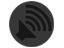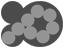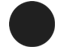B-L-A-C-K-O-P

 Saturday, December 29, 2007 Quartz Composer Patch : Borrow Alpha Component `/* Published by b-l-a-c-k-o-p.comCopyright (c) 2007-2009http://creativecommons.org/licenses/by-nc-sa/3.0/legalcode */` SAMPLE: QameraBondReloaded.qtzThis kernel extracts the alpha channel from the mask image, inverts it, and inserts the alpha channel in the result image. The source RGB values pass through unchanged.```kernel vec4 msBlackBorrowAlpha(sampler image, sampler mask){ vec4 pixel = sample(image, samplerCoord(image)); vec4 maxel = sample(mask, samplerCoord(mask)); pixel.a = 1.0-maxel.a; return pixel; } `````` Core Image/GLSL code Copyright (C) 2007-2009 b-l-a-c-k-o-p.com under Creative Commons license.Tags: Core Image | Quartz Composer | vj | macintosh By : Ms. Black Borrow Alpha Component 0 comments`````` ```
 Quartz Composer Patch : Brightness Mapping (RGB) `/* Published by b-l-a-c-k-o-p.comCopyright (c) 2007-2009http://creativecommons.org/licenses/by-nc-sa/3.0/legalcode */` SAMPLE: QameraSparklStars2.qtzThis code evaluates each pixel in the mask, extracts the brightness from it, and then adjusts the brightness of the source pixel by the amount of the mask brightness. The input_range value controls the amount of the effect.`kernel vec4 msBlackBrightnessMap(sampler image, sampler mask, float input_range){float range = clamp(input_range+0.1,0.0,1.0);float inverse_range = 1.0-range;float brightness; vec4 pixel = sample(image, samplerCoord(image)); vec3 maxel = sample(mask, samplerCoord(mask)); brightness = range*dot(maxel,maxel); brightness += inverse_range; pixel.r *= brightness; pixel.g *= brightness; pixel.b *= brightness; return clamp(pixel,0.0,1.0);}` By : Ms. Black Brightness Mapping (RGB) 0 commentsQuartz Composer Patch : Andy Warhol Style Silkscreen Filter `/* Published by b-l-a-c-k-o-p.comCopyright (c) 2007-2009http://creativecommons.org/licenses/by-nc-sa/3.0/legalcode */` SAMPLE: QameraPosterCamo.qtzThis Core Image kernel creates an Andy Warhol style silkscreen image. Andy used to take polaroids of people, generate a silkscreen mask with the blown up polaroid image, and then silkscreen the black outline over various other colors and so forth. He also hand-painted after silkscreening. Him and his factory of cheap laborers of love. This filter does a pretty good job of it.`kernel vec4 msBlackSilkscreen(sampler input_image, float input_threshold){vec2 center = samplerCoord(input_image);vec4 pixel = sample(input_image, center);float normal = dot(vec3(pixel),vec3(pixel));float grey = clamp(float(normal>input_threshold),0.0,1.0);float alpha = 1.0-grey; pixel.r = grey; pixel.g = grey; pixel.b = grey; pixel.a = grey; return pixel;}` By : Ms. Black Andy Warhol Style Silkscreen Filter 0 commentsFriday, December 28, 2007 Quartz Composer Patch : Contrast Mapping (YUV) `/* Published by b-l-a-c-k-o-p.comCopyright (c) 2007-2009http://creativecommons.org/licenses/by-nc-sa/3.0/legalcode */` SAMPLE: QameraSparklStars.qtzThis kernel extracts the overall brightness of each mask pixel, and applies that brightness level as a contrast adjustment to each source pixel in the input image.`kernel vec4 msBlackContrastMap(sampler image, sampler mask, float input_offset){const vec3 rgb_y = vec3(0.257, 0.504, 0.098);const vec3 rgb_u = vec3(-0.148, -0.291, 0.439);const vec3 rgb_v = vec3(0.439, -0.368, -0.071);const vec3 yuv_r = vec3(1.0000, 0.0000, 1.4022 );const vec3 yuv_g = vec3( 1.0000, -0.3457, -0.7145);const vec3 yuv_b = vec3(1.0000, 1.7710, 0.0000); vec4 pixel = sample(image, samplerCoord(image)); vec3 pel = pixel;float contrast = 1.0; vec3 maxel = sample(mask, samplerCoord(mask)); contrast = dot(maxel,maxel);float clamped_contrast = clamp(contrast+input_offset,0.0,1.0);vec3 pixel_yuv; pixel_yuv.x = dot(pel,rgb_y); pixel_yuv.y = dot(pel,rgb_u); pixel_yuv.z = dot(pel,rgb_v);float pixel_x = pixel_yuv.x; pixel_x *= clamped_contrast; pixel_yuv.x = pixel_x; pixel_yuv.y = pixel_yuv.y; pixel_yuv.z = pixel_yuv.z; pel.r = dot(pixel_yuv,yuv_r); pel.g = dot(pixel_yuv,yuv_g); pel.b = dot(pixel_yuv,yuv_b); return vec4(pel.r,pel.g,pel.b,pixel.a);}` By : Ms. Black Contrast Mapping (YUV) 0 commentsQuartz Composer Patch : Tint Mapping `/* Published by b-l-a-c-k-o-p.comCopyright (c) 2007-2009http://creativecommons.org/licenses/by-nc-sa/3.0/legalcode */` SAMPLE: QameraRainbow.qtzThis kernel takes a mask image, extracts the chromaticity from the mask, and applies the color to the source image without affecting the luma (brightness). You may want to front-load the input image with a contrast bump and/or desaturate the mask image slightly. Basically, this allows you to "steal" the color from an image without taking the brightness.`kernel vec4 msBlackTintMapping(sampler image, sampler mask){const vec3 rgb_y = vec3(0.257, 0.504, 0.098);const vec3 rgb_u = vec3(-0.148, -0.291, 0.439);const vec3 rgb_v = vec3(0.439, -0.368, -0.071);const vec3 yuv_r = vec3(1.0000, 0.0000, 1.4022 );const vec3 yuv_g = vec3( 1.0000, -0.3457, -0.7145);const vec3 yuv_b = vec3(1.0000, 1.7710, 0.0000); vec4 pixel = sample(image, samplerCoord(image)); vec3 pel = pixel; vec3 maxel = sample(mask, samplerCoord(mask));vec3 tint_yuv; tint_yuv.x = dot(maxel,rgb_y); tint_yuv.y = dot(maxel,rgb_u); tint_yuv.z = dot(maxel,rgb_v);vec3 pixel_yuv; pixel_yuv.x = dot(pel,rgb_y); pixel_yuv.y = dot(pel,rgb_u); pixel_yuv.z = dot(pel,rgb_v); pixel_yuv.y = tint_yuv.y; pixel_yuv.z = tint_yuv.z; pel.r = dot(pixel_yuv,yuv_r); pel.g = dot(pixel_yuv,yuv_g); pel.b = dot(pixel_yuv,yuv_b); return vec4(pel.r,pel.g,pel.b,pixel.a);}` By : Ms. Black Tint Mapping 0 commentsQuartz Composer Patch : Mask Extraction from Any Image `/* Published by b-l-a-c-k-o-p.comCopyright (c) 2007-2009http://creativecommons.org/licenses/by-nc-sa/3.0/legalcode */` SAMPLE: QameraSofterIris.qtzAs I was dissatisfied with the mask extraction/merging available in QC, we wrote this patch to be able to extract a matte, or alpha channel, from just about any source image. The kernel works by taking the dot product of the mask pixel, which ultimately is a normalized distance from black, and migrates that value into the alpha channel of the source image. This kernel works great, we use it everywhere.`kernel vec4 msBlackMergeAlphaFromGrey(sampler image, sampler mask){ vec4 pixel = sample(image, samplerCoord(image)); vec4 maxel = sample(mask, samplerCoord(mask)); float maskpel = clamp(0.333 * (maxel.r + maxel.g + maxel.b), 0.0, 1.0); pixel.a = maskpel; return pixel;}`If you need the inverse of the matte, either use this kernel, or run the image through Color Invert before extracting:`kernel vec4 msBlackMergeAlphaFromGrey(sampler image, sampler mask){ vec4 pixel = sample(image, samplerCoord(image)); vec4 maxel = sample(mask, samplerCoord(mask)); float maskpel = clamp(0.333 * (maxel.r + maxel.g + maxel.b), 0.0, 1.0); pixel.a = 1.0 - maskpel; return pixel;}` By : Ms. Black Mask Extraction from Any Image 0 commentsQuartz Composer Patch : Cheap Blur 3x3 `/* Published by b-l-a-c-k-o-p.comCopyright (c) 2007-2009http://creativecommons.org/licenses/by-nc-sa/3.0/legalcode */` A blur kernel (3x3 convolution, basically) cheap enough to run on !ARBfp hardware ("Quartz Extreme"), circa 2002.`/* cheap, as in !!arbfp cheap */kernel vec4 msBlackCheapBlur(sampler input_image, float input_radius){float clamped_radius = input_radius;float delta_x = 1.0 / (0.1+clamped_radius*0.5);float delta_y = 1.0 / (0.1+clamped_radius*0.5);vec2 center = samplerCoord(input_image);vec4 c = sample(input_image, center + vec2(-delta_x,-delta_y));vec4 bl = sample(input_image, center + vec2(-delta_x,-delta_y));vec4 l = sample(input_image, center + vec2(-delta_x, 0.0));vec4 tl = sample(input_image, center + vec2(-delta_x, delta_y));vec4 t = sample(input_image, center + vec2( 0.0, delta_y));vec4 ur = sample(input_image, center + vec2( delta_x, delta_y));vec4 r = sample(input_image, center + vec2( delta_x, 0.0));vec4 br = sample(input_image, center + vec2( delta_x, delta_y));vec4 b = sample(input_image, center + vec2( 0.0,-delta_y));vec4 blur = 0.25 * c + 0.125 * (l + t + r + b) + 0.0625 * (bl + tl + ur + br); return blur;}` By : Ms. Black Cheap Blur 3x3 0 commentsQuartz Composer Patch : Blur Mapping `/* Published by b-l-a-c-k-o-p.comCopyright (c) 2007-2009http://creativecommons.org/licenses/by-nc-sa/3.0/legalcode */` SAMPLE: QameraWetter.qtzThis kernel takes a mask image, and uses the brightness of the mask pixel as the input to a 3x3 blur kernel. This kernel has proved useful in creating "water on glass" effects. It's derived from msBlackCheapBlur, which is listed elsewhere on this page. One of the reasons that we developed cheap blur (actually, ported from shader fragments in cyberspace) is because Core Image gaussian blur seems to be invoking the software OpenGL renderer on the Intel MacBooks. The performance hit is noticeable, as is the increase in memory bandwidth usage. Mr. Black pointed this out, I am simply relaying the info.UPDATE: This blur mapping kernel is also exceeding the fragment program limits on the MacBook. More research underway...`kernel vec4 msBlackBlurMap(sampler input_image, sampler input_mask_image){vec3 maxel = sample(input_mask_image, samplerCoord(input_mask_image));float clamped_radius = dot(maxel,maxel);float delta_x = 1.0 / (0.1+clamped_radius*0.5);float delta_y = 1.0 / (0.1+clamped_radius*0.5);vec2 center = samplerCoord(input_image);vec4 c = sample(input_image, center + vec2(-delta_x,-delta_y));vec4 bl = sample(input_image, center + vec2(-delta_x,-delta_y));vec4 l = sample(input_image, center + vec2(-delta_x, 0.0));vec4 tl = sample(input_image, center + vec2(-delta_x, delta_y));vec4 t = sample(input_image, center + vec2( 0.0, delta_y));vec4 ur = sample(input_image, center + vec2( delta_x, delta_y));vec4 r = sample(input_image, center + vec2( delta_x, 0.0));vec4 br = sample(input_image, center + vec2( delta_x, delta_y));vec4 b = sample(input_image, center + vec2( 0.0,-delta_y));vec4 blur = 0.25 * c + 0.125 * (l + t + r + b) + 0.0625 * (bl + tl + ur + br); return blur;}` By : Ms. Black Blur Mapping 0 commentsQuartz Composer Patch : Image Registration: YUV, Rectangular (dx,dy) `/* Published by b-l-a-c-k-o-p.comCopyright (c) 2007-2009http://creativecommons.org/licenses/by-nc-sa/3.0/legalcode */` SAMPLE: QameraBetterPopArt5.qtzThis is similar to the ARGB registration kernels, but it works in YUV color space, where the brightness is already separated from the chromaticity. Thus, when you de-register, the deregistered color is more closely related to the center.`kernel vec4 msBlackRectRegistrationYUV(sampler input_image, vec2 u_delta, vec2 v_delta){const vec3 rgb_y = vec3(0.257, 0.504, 0.098);const vec3 rgb_u = vec3(-0.148, -0.291, 0.439);const vec3 rgb_v = vec3(0.439, -0.368, -0.071);const vec3 yuv_r = vec3(1.0000, 0.0000, 1.4022 );const vec3 yuv_g = vec3( 1.0000, -0.3457, -0.7145);const vec3 yuv_b = vec3(1.0000, 1.7710, 0.0000);vec2 center = samplerCoord(input_image);vec4 pixel = sample(input_image, center);vec3 pel = pixel;vec3 u_pixel = sample(input_image, center + u_delta);vec3 v_pixel = sample(input_image, center + v_delta);vec3 pixel_yuv; pixel_yuv.x = dot(pel,rgb_y); pixel_yuv.y = dot(u_pixel,rgb_u); pixel_yuv.z = dot(v_pixel,rgb_v); pixel.r = dot(pixel_yuv,yuv_r); pixel.g = dot(pixel_yuv,yuv_g); pixel.b = dot(pixel_yuv,yuv_b); return pixel;}` By : Ms. Black Image Registration: YUV, Rectangular (dx,dy) 0 commentsQuartz Composer Patch : Image Registration: ARGB Rectangular (dx,dy) `/* Published by b-l-a-c-k-o-p.comCopyright (c) 2007-2009http://creativecommons.org/licenses/by-nc-sa/3.0/legalcode */` SAMPLE: QameraBetterPopArt2.qtz`/* de-register the RGB color planes of an image by r_delta,g_delta,b_delta */kernel vec4 msBlackRectangularRegistration(sampler input_image, vec2 r_delta, vec2 g_delta, vec2 b_delta){vec2 center = samplerCoord(input_image);vec4 pixel = sample(input_image, center);vec4 red = sample(input_image, center + r_delta);vec4 green = sample(input_image, center + g_delta);vec4 blue = sample(input_image, center + b_delta); pixel.r = red.r; pixel.g = green.g; pixel.b = blue.b; return pixel;}` By : Ms. Black Image Registration: ARGB Rectangular (dx,dy) 0 commentsQuartz Composer Patch : Image Registration: ARGB Polar(radius,theta) `/* Published by b-l-a-c-k-o-p.comCopyright (c) 2007-2009http://creativecommons.org/licenses/by-nc-sa/3.0/legalcode */` SAMPLE: QameraBetterPopArt3.qtzThis Core Image page 'de-registers' the color planes of an image. A common technique among graphic designers when running traditional print jobs. Of course, with PhotoShop, nobody does it at the printer anymore.`float as_radians(const float in_degrees){ return in_degrees * (3.14597/180.0);}vec2 from_polar(const float in_radius, const float in_theta){float x = in_radius * sin(as_radians(in_theta));float y = in_radius * cos(as_radians(in_theta)); return vec2(x,y);}kernel vec4 msBlackPolarRegistration(sampler input_image, float radius_r, float angle_r, float radius_g, float angle_g, float radius_b, float angle_b){vec2 r_delta = from_polar(radius_r, angle_r);vec2 g_delta = from_polar(radius_g, angle_g);vec2 b_delta = from_polar(radius_b, angle_b);vec2 center = samplerCoord(input_image);vec4 pixel = sample(input_image, center);vec4 red = sample(input_image, center + r_delta);vec4 green = sample(input_image, center + g_delta);vec4 blue = sample(input_image, center + b_delta); pixel.r = red.r; pixel.g = green.g; pixel.b = blue.b; return pixel;}` By : Ms. Black Image Registration: ARGB Polar(radius,theta) 0 commentsQuartz Composer Patch : Introducing Ms. Black's QC Kitchen, home of VJ FOOD `/* Published by b-l-a-c-k-o-p.comCopyright (c) 2007-2009http://creativecommons.org/licenses/by-nc-sa/3.0/legalcode */` Over the holiday I had a chance to kick back and write some GLSL Kernels for our new Qamera FX. We're publishing these kernels/filters under a Creative Commons license. Non-commercial, share and share alike, with attribution. If you're a VJ and making custom patches for your own performance, feel free to use these. The non-commercial aspect really applies to inclusion in a commercial product.Here are the kernels and the descriptions of what each does. You can find examples of their use within the .QTZ patches in Qamera. Qamera 1.x places it's Quartz Composer patches in a non-localized ~/Library/Compositions folder. This is Ms. Black's QC Kitchen, a place where VJs can 'help themselves' to the VJ FOOD ;) By : Ms. Black Introducing Ms. Black's QC Kitchen, home of VJ FOOD 0 commentsSITE MAP
BLACKOP
PRESSDotMatrix
CamCamX
GrabberRaster
Q@mera
VirtualEyez
3D
StoreTutorials
DIY Greenscreen
3D Webcam Mac
USB 3D webcam
BlackenedPixels
CatEye
CamCamX Blog
CatEye Blog
DotMatrix Blog
GrabberRaster Blog
Qamera Blog
Quicky
AsSceneOnTV
Watermarks
Watermarks Blog
PDF Watermarking Mac
PDFBatchBuild
PDF Combine Mac
Combine PDF Files
Create iTunes Booklet
Share Your Pictures
Washington DC
Universal Binary
Cocoa Programming
Cocoa Software
Screen Capture
Screen Grab Mac
iSight software
WebCam
iPhoto
Photoshop
MacBook Camera
iMac Camera
CamCamX compatibility
Fake WebCam Mac
VJ Software Mac
Video Mixer Mac
Live Video Switcher
Apple Webcam Effects
Webcam Video Effects
Free iChat Video Effects
Skype Video Effects Mac
Video Effects Stickam
iSight Virtualizer Mac
Webcast Streaming Mac
Best Screencast Mac
Desktop Streaming Mac
Screencast Streaming Mac
Quartz Composer
Quartz Composer Plugins
Quartz Composer Patches
Mogulus Video FX
Video Effects Ustream
Operator11 Video FX
Polaroid Camera Emulator
No recording device found
Photo Booth cannot open the camera